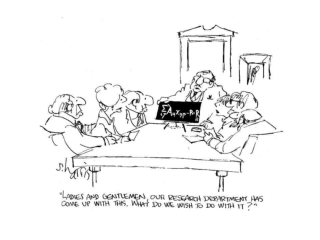DownloadDownload PresentationProblem 31

# Problem 31

Télécharger la présentation## Problem 31

- - - - - - - - - - - - - - - - - - - - - - - - - - - E N D - - - - - - - - - - - - - - - - - - - - - - - - - - -
##### Presentation Transcript

1. Problem 31 Find the moments of inertia about the x & y axes: m = 1.8 kg, M = 3.1 kg

2. Problem 40 m each blade = 160 kg. Moment of inertia I? Starts from rest, torque τto get ω = 5 rev/s (10πrad/s) in t = 8 s?

3. Example 8-11 M = 4 kg, FT = 15 N Frictional torque:τfr = 1.1 m N t = 0, ω0 = 0; t = 3 s, ω = 30 rad/s I = ? Calculate the angular acceleration: ω = ω0+ αt, α = (ω - ω0)/t = 10 rad/s2 N’s 2nd Law:∑τ = Iα FTR - τfr = Iα  I = [(15)(0.33) -1.1]/10 I = 0.385 kg m2

4. Example 8-12 The same pulley, connected to a bucket of weight mg = 15 N (m = 1.53 kg). M = 4 kg I = 0.385 kg m2; τfr = 1.1 m N a)α = ? (pulley) a = ?(bucket) b)t = 0, at rest. t = 3 s, ω = ? (pulley) v = ? (bucket) a 

5. Translation-Rotation Analogues & Connections Translation Rotation Displacement x θ Velocity v ω Acceleration a α Force (Torque) F τ Mass (moment of inertia) m I Newton’s 2nd Law:∑F = ma ∑τ = Iα Kinetic Energy (KE) (½)mv2 ? CONNECTIONS v = rω, atan= rα, aR = (v2/r) = ω2 r τ = rF, I = ∑(mr2)

6. Section 8-7: Rotational Kinetic Energy • Translational motion (Ch. 6): (KE)trans = (½)mv2 • Rigid body rotation, angular velocity ω.Rigid Every point has the same ω.Body is made of particles, masses m. • For each m at a distance r from the rotation axis: v = rω. The Rotational KE is: (KE)rot = ∑[(½)mv2] = (½)∑(mr2ω2) = (½)∑(mr2)ω2 ω2 goes outside the sum, since it’s the same everywhere in the body • As we just saw, the moment of inertia, I  ∑(mr2)  (KE)rot = (½)Iω2 (Analogous to (½)mv2)

7. Translation-Rotation Analogues & Connections Translation Rotation Displacement x θ Velocity v ω Acceleration a α Force (Torque) F τ Mass (moment of inertia) m I Newton’s 2nd Law ∑F = ma ∑τ = Iα Kinetic Energy (KE) (½)mv2 (½)Iω2 CONNECTIONS v = rω, atan= rα, aR = (v2/r) = ω2 r τ = rF, I = ∑(mr2)

8. Sect. 8-7: Rotational + Translational KE • Rigid body rotation:(KE)rot = (½)Iω2 • Now, consider a rigid body, mass M, rotating (angular velocity ω) about an axis through the CM. At the same time, the CM is translating with velocity vCM • Example, a wheel rolling without friction. For this, we saw earlier that vCM = rω. The KE now has 2 parts:(KE)trans & (KE)rot  Total KE = translational KE + rotational KE KE = (KE)trans + (KE)rot or KE = (½)M(vCM)2 + (½)ICMω2 where: ICM = Moment of inertia about an axis through the CM

9. Example 8-13 A sphere rolls down an incline (no slipping or sliding). KE+PE conservation: (½)Mv2 + (½)Iω2 + MgH = constant, or (KE)1 +(PE)1 = (KE)2 + (PE)2 where KE has 2 parts!! (KE)trans = (½)Mv2 (KE)rot = (½)Iω2  v = 0, ω = 0 v = ?  y = 0

10. Conceptual Example 8-14:Who Wins the Race? v increases as I decreases! Demonstration! MgH = (½)Mv2 + (½)ICMω2 Gravitational PE is Converted to Translational + Rotational KE! Hoop: ICM = MR2 Cylinder: ICM = (½)MR2 Sphere: ICM = (2/5)MR2(also, v = ωR)

11. Friction: Necessary for objects to roll without slipping. Example: work done by friction hasn’t been included (used KE +PE =const). WHY? Because Ffr Motion  Ffrdoes no work!